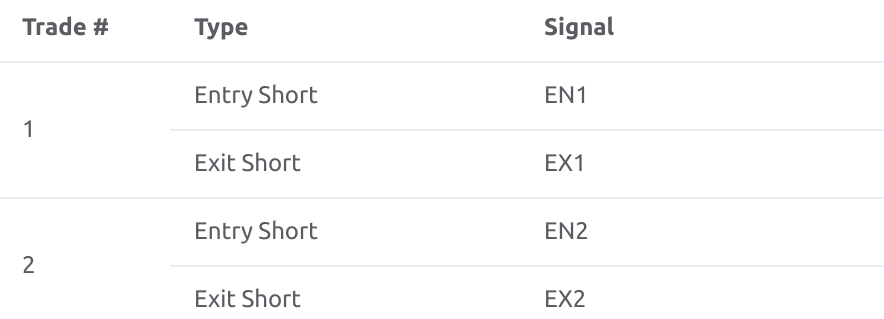# My strategy ignores the ‘from_entry’ argument while closing entries

The strategy.exit() function allows you to exit a specific entry using the from_entry parameter.

If the strategy has more than one entry (pyramiding), the order in which the market positions are closed in the trade list of the strategy tester may be unclear.

By default, the first exit order sent by a strategy closes the oldest entry order. Then the next exit order closes the next oldest entry, and so on. Essentially, the strategy closes trades based on the first in, first out  (FIFO) principle.

In the following example we try using the from_entry argument to connect strategy.exit calls to specific entries:

``````//@version=4
strategy("close_entries_rule_example", overlay=true, pyramiding = 2)

strategy.entry("EN2", strategy.short, when= bar_index == 20650)
strategy.entry("EN1", strategy.short, when= bar_index == 20652)

if bar_index == 20655
strategy.exit(id = "EX1", from_entry = "EN1", profit = 1, loss = 1)

if bar_index == 20657
strategy.exit(id = "EX2", from_entry = "EN2", profit = 1, loss = 1)``````

First, strategy.entry opens a short position EN2 at the bar with the index of 20650, and EN1 enters two bars after that.

When bar_index is equal to 20655, a corresponding strategy.exit() function with id = EX1 is going to exit entry EN1, and  EX2 will exit EN2 two bars after.

But the strategy tester results look like this:The first trade with the id EN2 is closed by the exit order EX1, and EN1 is closed by EX2, even though it was coded that EN2 should be closed by EX2 at the bar 20657. So the first exit order has closed the first entry order, and the second exit order has closed the second entry. That's classic FIFO behavior.

To make it possible for the strategy to close orders in any sequence, the close_entries_rule argument of the strategy() function should be set to "ANY" (instead of the default “FIFO”), e.g:

``//@version=4strategy(..., close_entries_rule="ANY", ...)``

With this "ANY" value the strategy can determine which exit order closes which specific position. (Note that "FIFO" is implicitly set as default even when close_entries_rule argument is not specified).

With the strategy() call changed to

``strategy("close_entries_rule_example", close_entries_rule="ANY",  overlay=true, pyramiding = 2)``

the strategy tester results will be different:In this case, the (chronologically) first exit order EX1 closed the second entry EN1, and the second exit closed the first entry.